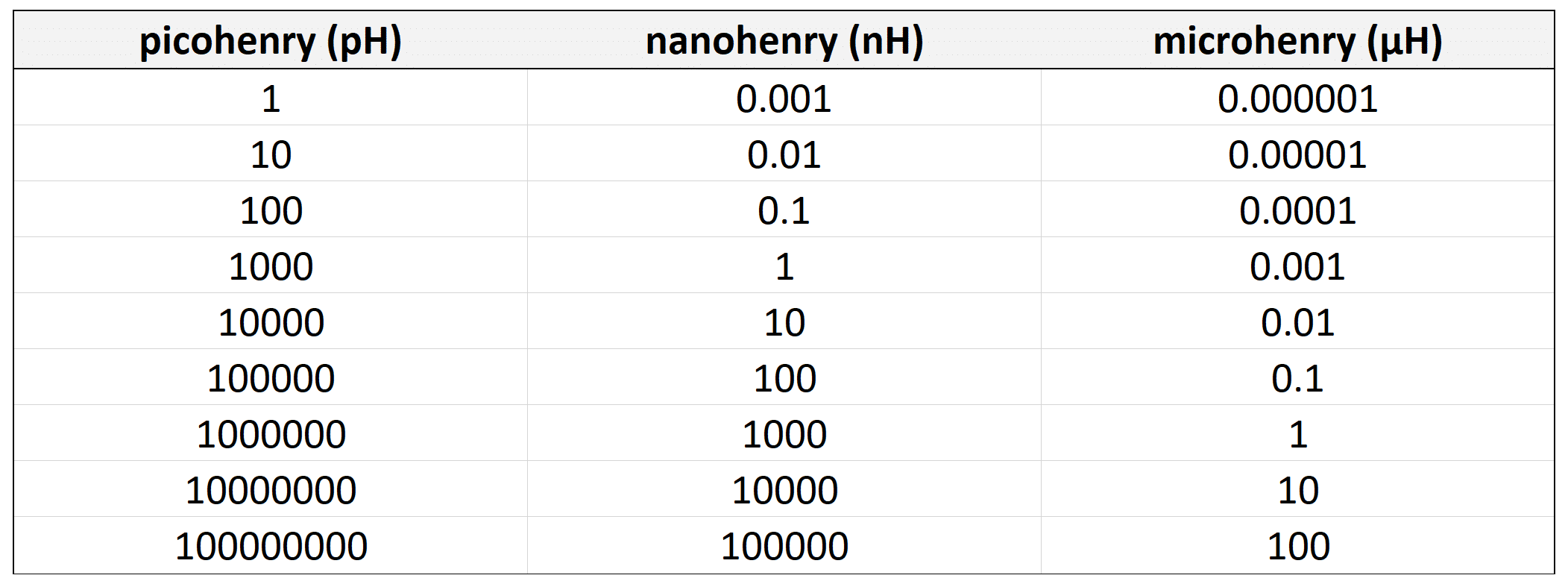# Inductance Converter

henry:
H
kilohenry:
kH
millihenry:
mH
microhenry:
µH
nanohenry:
nH
picohenry:
pHhenry:
H
kilohenry:
kH
millihenry:
mH
microhenry:
µH
nanohenry:
nH
picohenry:
pHInductance, in electromagnetism and electronics, is a basic electrical measurement along with resistance and capacitance. It is the ability of an inductor to store energy created by the current flowing through it. This flow of electric current creates a magnetic field. The strength of that magnetic field depends on the magnitude of the current. Inductors generate opposing voltage that is proportional to the rate of change in the current in a circuit.

Conversions can sometimes be easy if you are talking about the same unit such as micrograms to grams. Other times conversions can be much more difficult like converting between metric and imperial units such as kilograms to ounces. Suntsu has created a weight unit converter to assist you. Our converter can help you convert between the following units:

Metric Ton (t)
Kilogram (kg)
Gram (g)
Milligram (mg)
Microgram (µg)

US Ton (ST)
Imperial Ton (LT)
Pounds (lb)
Ounces (oz)
Stones (st)